Question

7. Water flows through a pipe of diameter 1.08m at a velocity of 2m/s. If someone puts a nozzle on the end of the pipe, reducing the diameter to 0.35m, at what speed will the water exit the pipe?.

10. A pipe with a radius of 50 cm has water flowing at 5 m/s. If water goes into a new pipe with radius 33 cm, how much will the pressure decrease?

11. A large dam has a hole that is 56 meters from the top. If the water in the reservoir is depending at a rate of 0.33 m/s, how fast is the water exiting the hole?

12. A hand pumped water gun is held at height of 1.3 m above the ground. The water lands 8.4 meters away. What is the exit velocity of the stream? What is the gauge pressure of the gun's reservoir, assuming the velocity of the water is zero and doesn't change height before leaving the reservoir?

7) Using the continuity equation we have

A1v1 = A2v2

pi/4*1.08^2 * 2 = pi/4*0.35^2*v2

v2 = 19.04 m/s

exit speed is 19.04 m/s

10) A1v1 = A2v2

pi*0.5^2*5^2 = pi*0.33^2*v2

v2 = 11.47 m/s

Using the Bernoulli's equation we have

P1 + 0.5pv1^2 = P2 + 0.5pv2^2

P1-P2 = 0.5*p*(v2^2 - v1^2) = 0.5*1000*(11.47^2 - 5^2) = 53377.1 N/m^2

#### Earn Coins

Coins can be redeemed for fabulous gifts.

Similar Homework Help Questions
• ### A 2.5 cm diameter PVC water pipe brings city water into a home at a speed...

A 2.5 cm diameter PVC water pipe brings city water into a home at a speed of 0.038 m/s. The city water pipe is 45 cm below ground and the water outlet outside the house is 0.80 meters above ground. The exit velocity from the hose is 0.12 m/s exiting from a 2.5 cm diameter circular nozzle. What is city water pressure?

• ### Water flows at speed of 6 m/s through a horizontal pipe of diameter 3.5 cm. The...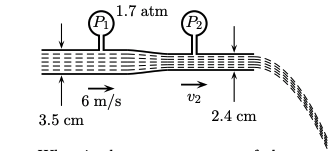Water flows at speed of 6 m/s through a horizontal pipe of diameter 3.5 cm. The gauge pressure P1 of the water in the pipe is 1.7 atm. A short segment of the pipe is constricted to a smaller diameter of 2.4 cm . What is the gauge pressure of the water flowing through the constricted segment? Atmospheric pressure is 1.013 × 10^5 Pa. The density of water is 1000 kg/m^3 . The viscosity of water is negligible. Answer in...

• ### A flow nozzle equipped with a differential pressure gage is used to measure the flow rate of water at 10°C (p 9997 kg/m3 and p 1.307 x 10-3 kg/m s) through a 3-cm-diameter horizontal pipe. The nozzle...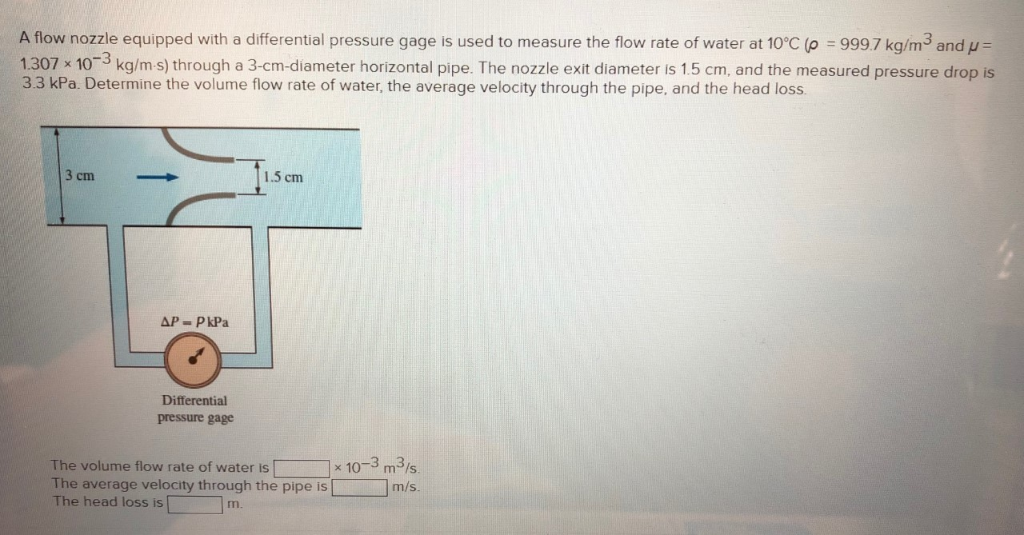A flow nozzle equipped with a differential pressure gage is used to measure the flow rate of water at 10°C (p 9997 kg/m3 and p 1.307 x 10-3 kg/m s) through a 3-cm-diameter horizontal pipe. The nozzle exit diameter is 1.5 cm, and the measured pressure drop is 3.3 kPa. Determine the volume flow rate of water, the average velocity through the pipe, and the head loss 1.5 cm Differential pressure gage -3 m3(s m/s The volume flow rate of...

• ### Water at 20°C flows at 0.08 m3/s through a pipe and is metered by a 12...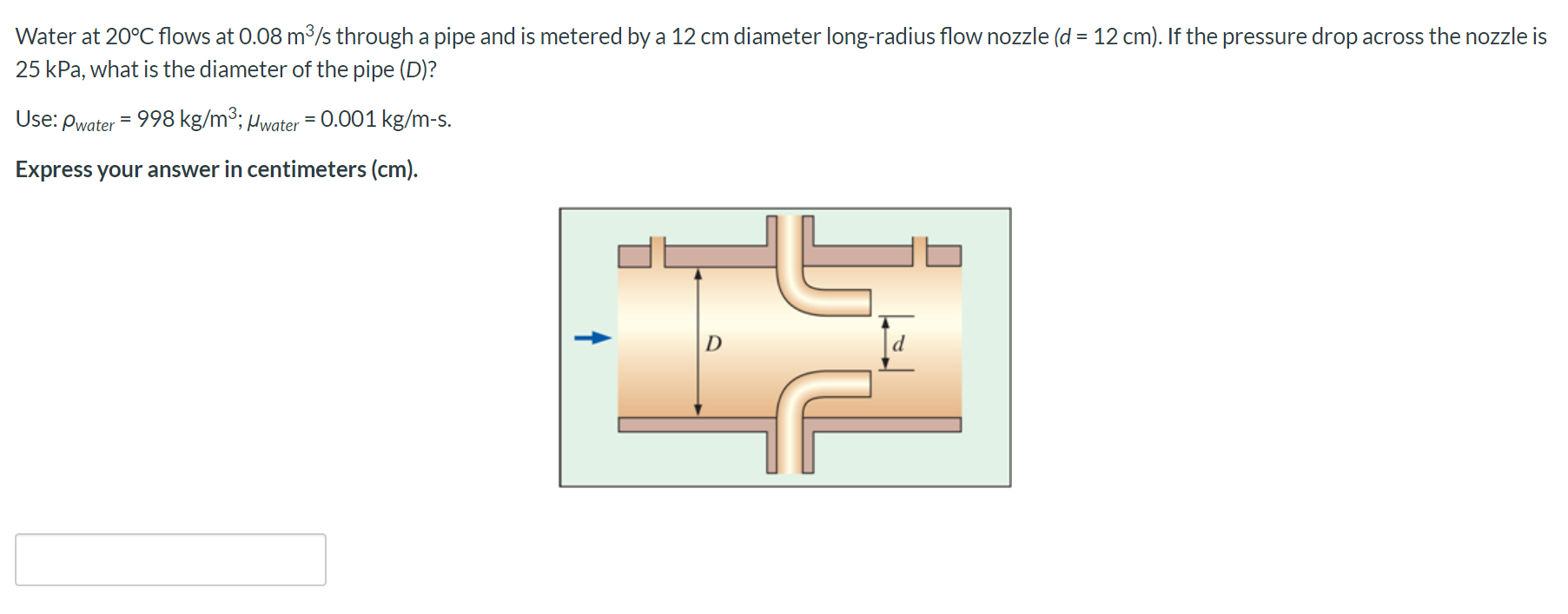Water at 20°C flows at 0.08 m3/s through a pipe and is metered by a 12 cm diameter long-radius flow nozzle (d = 12 cm). If the pressure drop across the nozzle is 25 kPa, what is the diameter of the pipe (D)? Use: Pwater = 998 kg/m3; Hwater = 0.001 kg/m-s. Express your answer in centimeters (cm). D

• ### 1) 2) 3) Oil flows through an 8-inch diameter pipe with a velocity of 18 ft/s....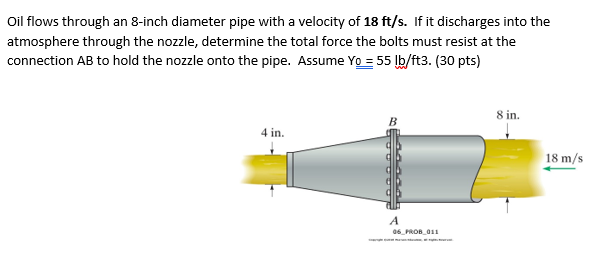1) 2) 3) Oil flows through an 8-inch diameter pipe with a velocity of 18 ft/s. If it discharges into the atmosphere through the nozzle, determine the total force the bolts must resist at the connection AB to hold the nozzle onto the pipe. Assume Yo = 55 lb/ft3. (30 pts) 8 in. 4 in. 18 m/s 06 PROB011 Research is being conducted on a medical specimen. They are trying to determine the pressure drop in fluid carrying vessels. If...

• ### (c) Water flows through a pipe of diameter 0.05 m at a speed of 1.5 m/s....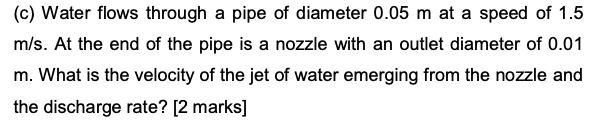(c) Water flows through a pipe of diameter 0.05 m at a speed of 1.5 m/s. At the end of the pipe is a nozzle with an outlet diameter of 0.01 m. What is the velocity of the jet of water emerging from the nozzle and the discharge rate? [2 marks]

• ### Water flow in a pipe and then exit through a bended nozzle as shown in Figure...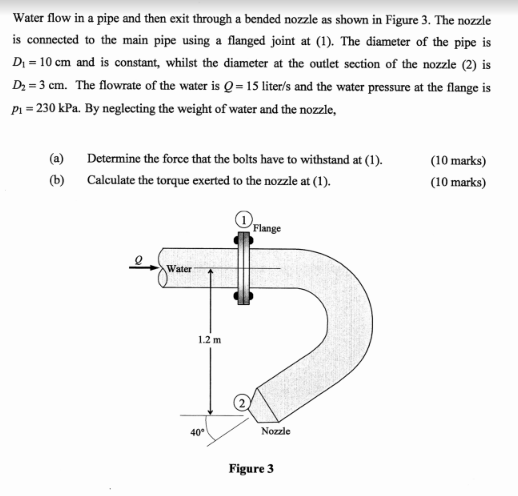Water flow in a pipe and then exit through a bended nozzle as shown in Figure 3. The nozzle is connected to the main pipe using a flanged joint at (1). The diameter of the pipe is D1 10 cm and is constant, whilst the diameter at the outlet section of the nozzle (2) is D2 3 em. The flowrate of the water is Q = 15 liter/s and the water pressure at the flange is Pi 230 kPa. By...

• ### 8. (15 points) Water flows through a 3-in diameter pipe with a velocity of 25 ft/s...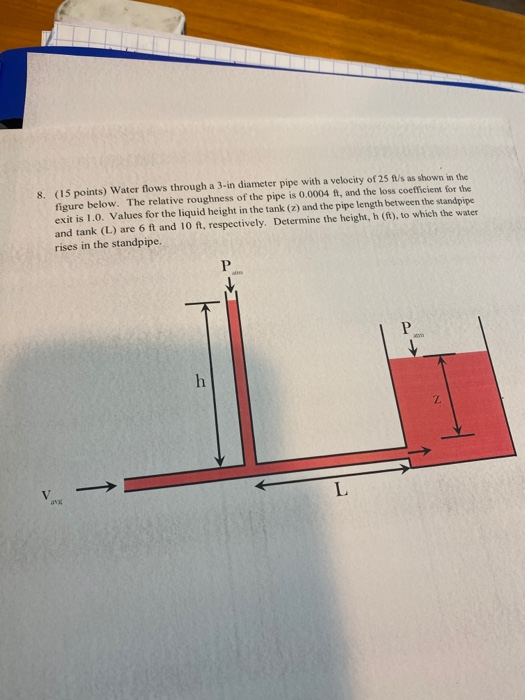8. (15 points) Water flows through a 3-in diameter pipe with a velocity of 25 ft/s as shown in the figure below. The relative roughness of the pipe is 0.0004 ft, and the loss coefficient for the exit is 1.0. Values for the liquid height in the tank (z) and the pipe length between the standpipe and tank (L) are 6 ft and 10 ft, respectively. Determine the height, h (ft), to which the water rises in the standpipe. P...

• ### Water flows from a fire truck through a hose that is 11.7 cm in diameter and...

Water flows from a fire truck through a hose that is 11.7 cm in diameter and has a nozzle that is 2.0 cm in diameter. When water leaves the nozzle, it has a speed of 20 m/s. Determine the minimum gauge pressure in the truck's water tank.

• ### 8. (15 points) Water flows through a 2-in diameter pipe with a velocity of 15 ft/s...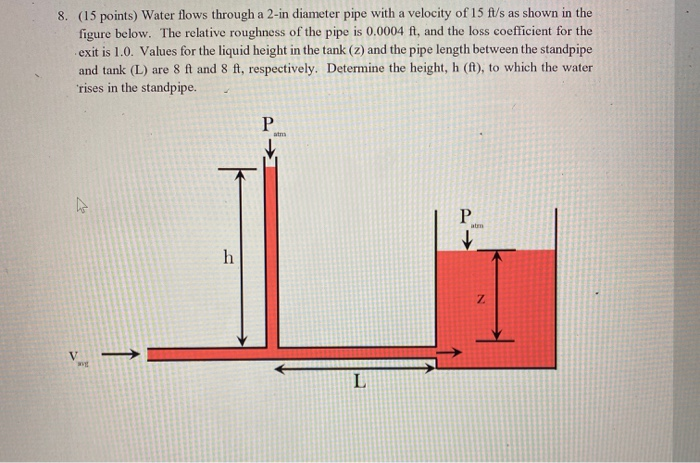8. (15 points) Water flows through a 2-in diameter pipe with a velocity of 15 ft/s as shown in the figure below. The relative roughness of the pipe is 0.0004 ft, and the loss coefficient for the exit is 1.0. Values for the liquid height in the tank (2) and the pipe length between the standpipe and tank (L) are 8 ft and 8 ft, respectively. Determine the height, h (ft), to which the water rises in the standpipe. P...